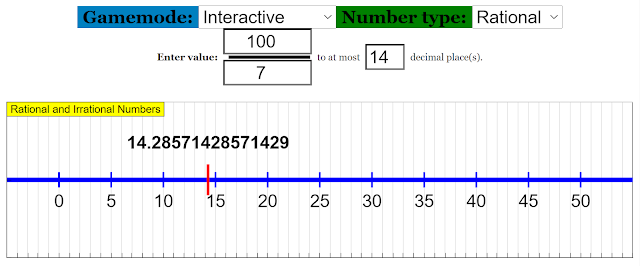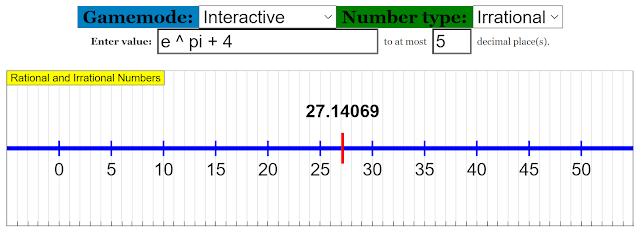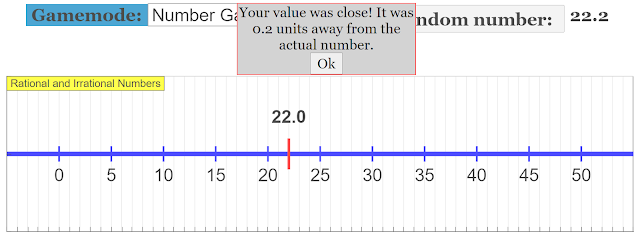{source}

<?phprequire_once JPATH_SITE.'/TTcustom/TT_contentparser.php';$$parameters = array("topicname" => "math","modelname" => "ejss_model_rationalNumbers2");echo generateSimHTML($$parameters, "EJSS");
?>
{/source}

[text]

# Interactive Tool for Visualising Rational and Irrational Numbers 2021 JavaScript HTML5 Applet

The link to the interactive can be found here.

## Description

This is a simple interactive to help students visualise rational and irrational numbers when placed on a number line. For rational numbers, they can manipulate the numerator and denominator to form the desired fraction. They can also change the number of decimal places displayed to gain a better understanding of repeating decimal values.Interactive displaying rational numbers
For irrational numbers, users are allowed to input basic mathematical expressions using common symbols such as + (plus), - (minus), * (times), / (divide) and ^ (power). Moreover, students can input common irrational numbers such as e and pi to facilitate their learning.Interactive displaying irrational values

This interactive also comes with a simple number game. Students can generate a random number, after which they can drag the red indicator on the number line to locate the value. A "Check answer" button is provided to help students determine how close their answer is to the desired value."Check answer" dialog showing how close the student's answer is

The link to the interactive can be found here.

Research

[text]

[text]

[text]